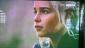# Elimination of Arbitrary Constant

## Eliminate the Arbitrary Constants

Please do leave a solution so I can study it, thank you!

Obtain the differential equation by eliminating the arbitrary constants.
1. Cxsiny+x^(2)y=Cy
2. y=Ae^(-3x)-Be^(2x)+Cx^3
3. Ay=e^(Bx^2)

## Differential equation: Eliminate the arbitrary constant from $y=c_1e^{5x}+c_2x+c_3$

y=c1e^5x+c2x+c3## Differential Equation: Eliminate $C_1$, $C_2$, and $C_3$ from $y=C_1e^x+C_2e^{2x}+C_3e^{3x}$

How to eliminate arbitrary constants
y=C1e^x+C2e^2x+C3e^3x

thank you :)

## Elimination of arbitrary constants: $y = Ae^{ax} \cos (bx) + Be^{ax} \sin (bx)$

Im having a difficulty solving this problem y = Aeaxcos(bx)+Beaxsin(bx) where a and b are parameters. Can anyone show me how to solve this ?## Differential Equation: Eliminate $c_1$ and $c_2$ from $y = c_1 e^x + c_2 xe^x$

eliminate the arbitrary constant:

y=c1ex + c2xex

y'=c1ex + c2(xex + ex)
y''=c1ex + c2(xex + 2ex)

by comparing eq.1 and eq,2 = eq,4
by comparing eq.2 and eq.3 = eq.5

by comparing eq.4 and eq.5 = y'' + 2y - 3y'

am i doing it right??